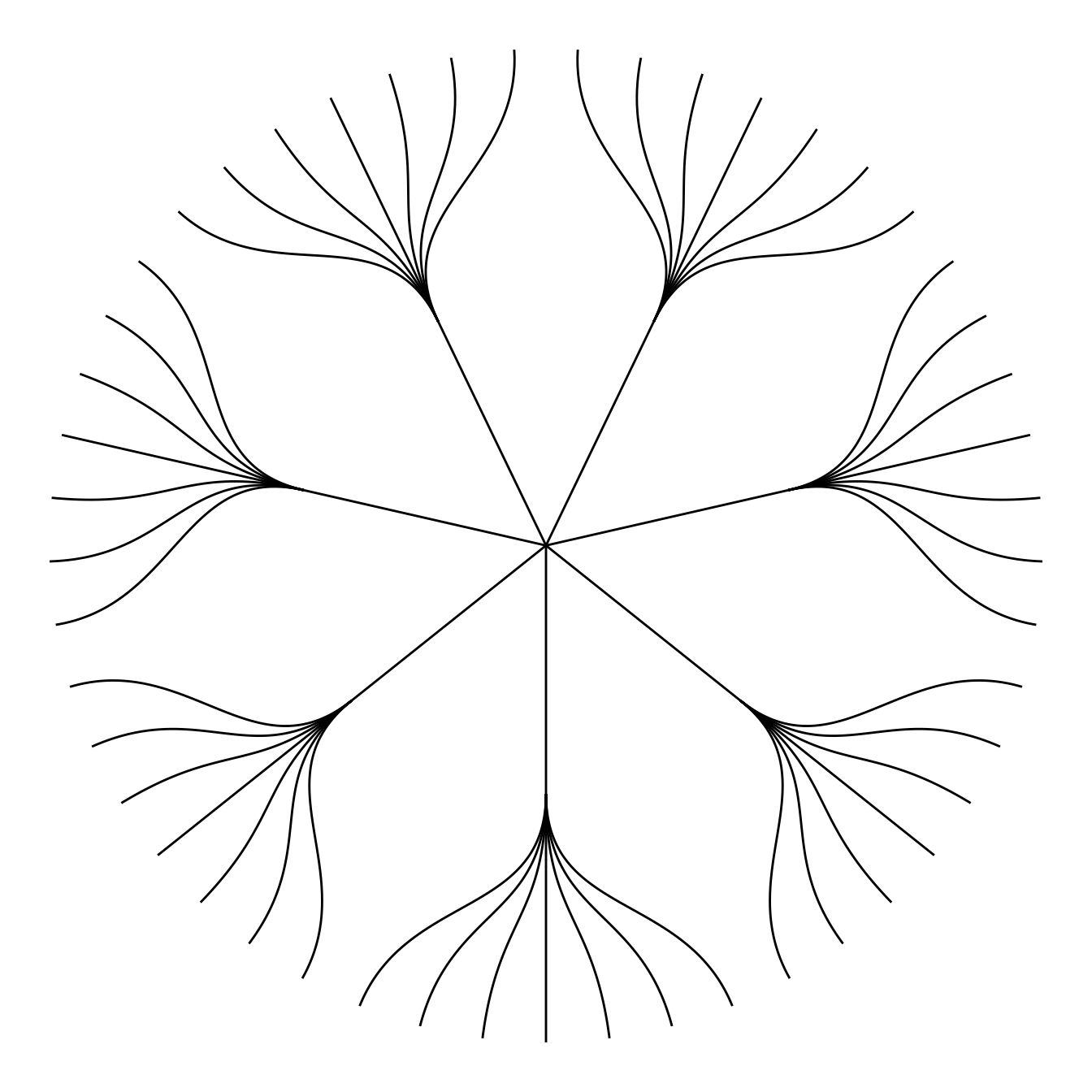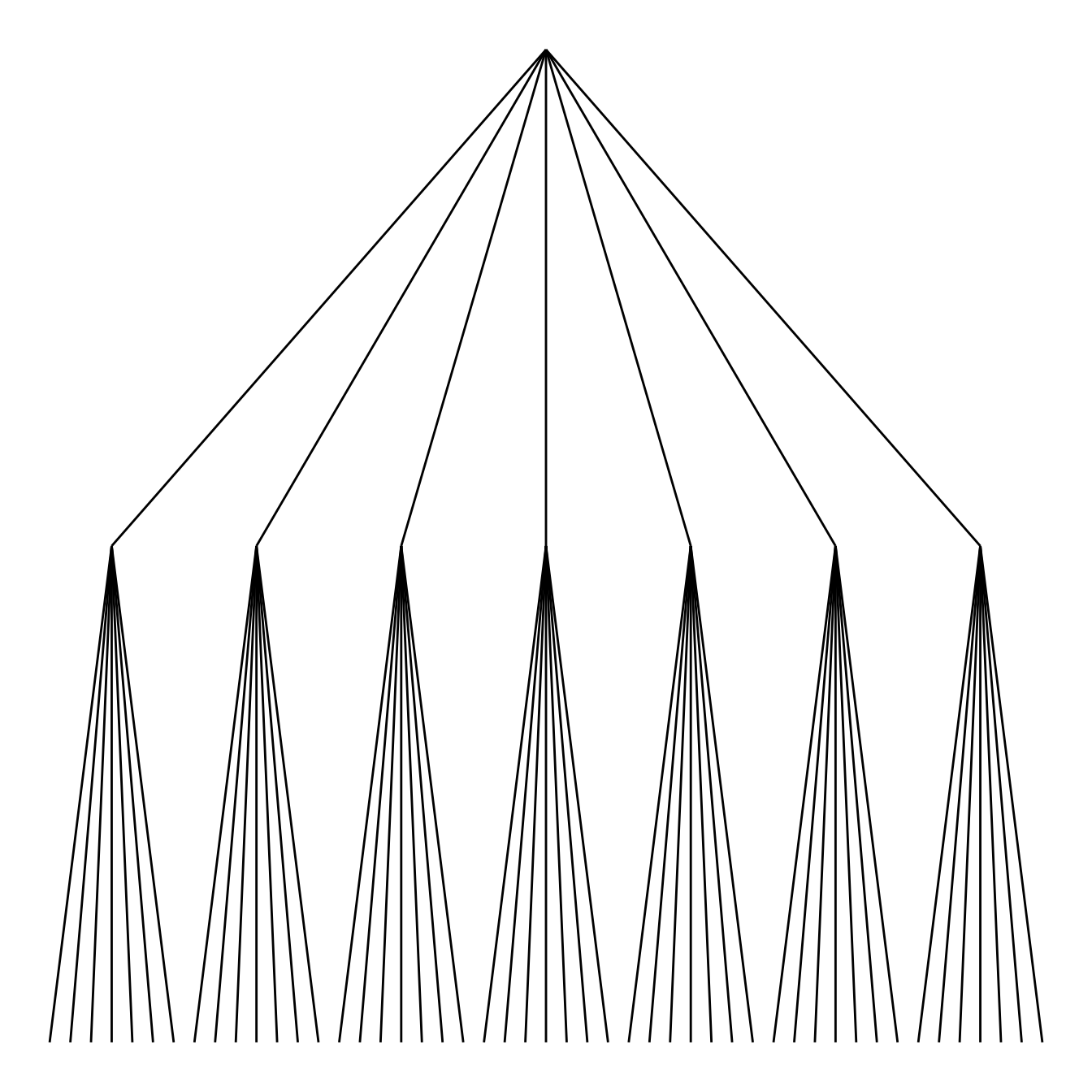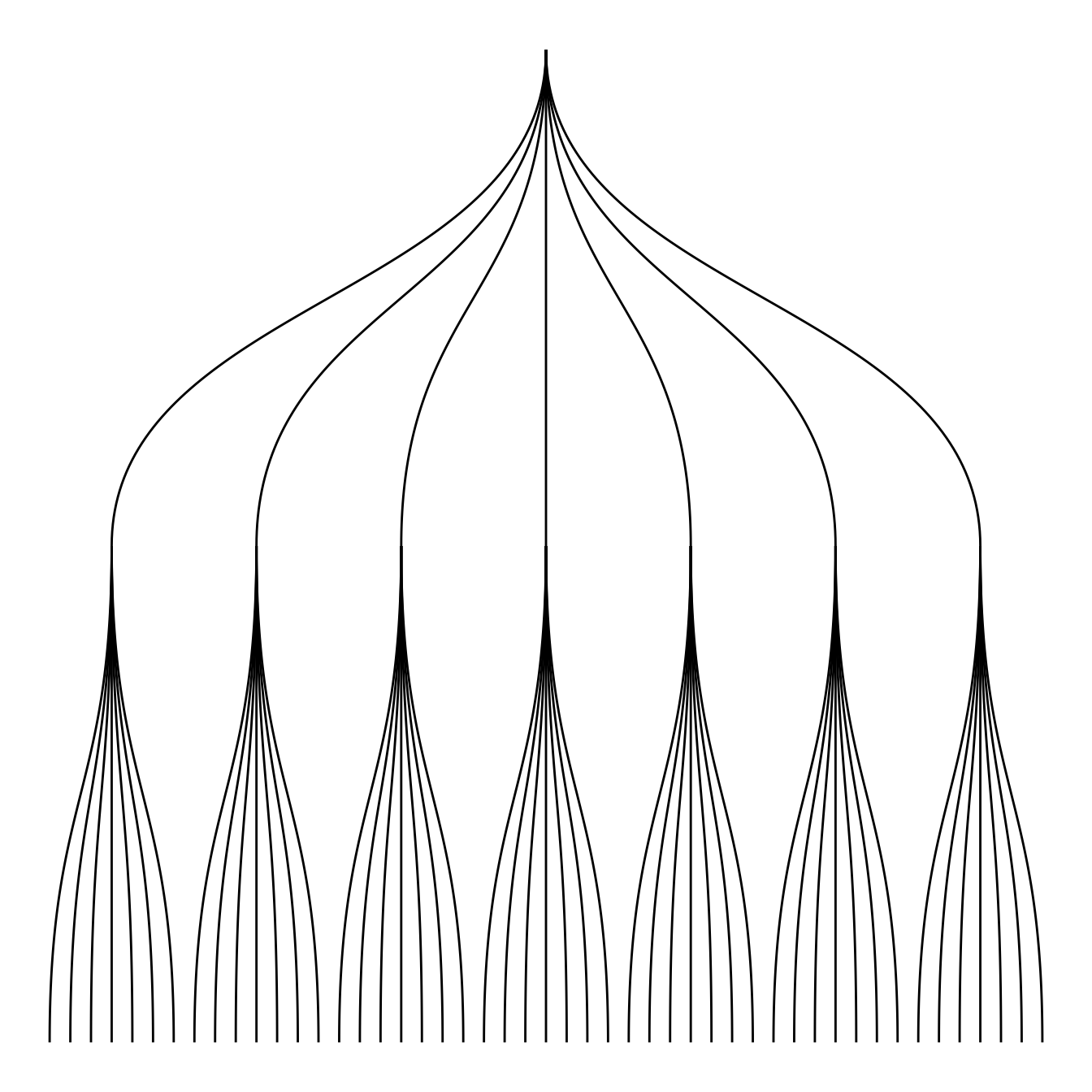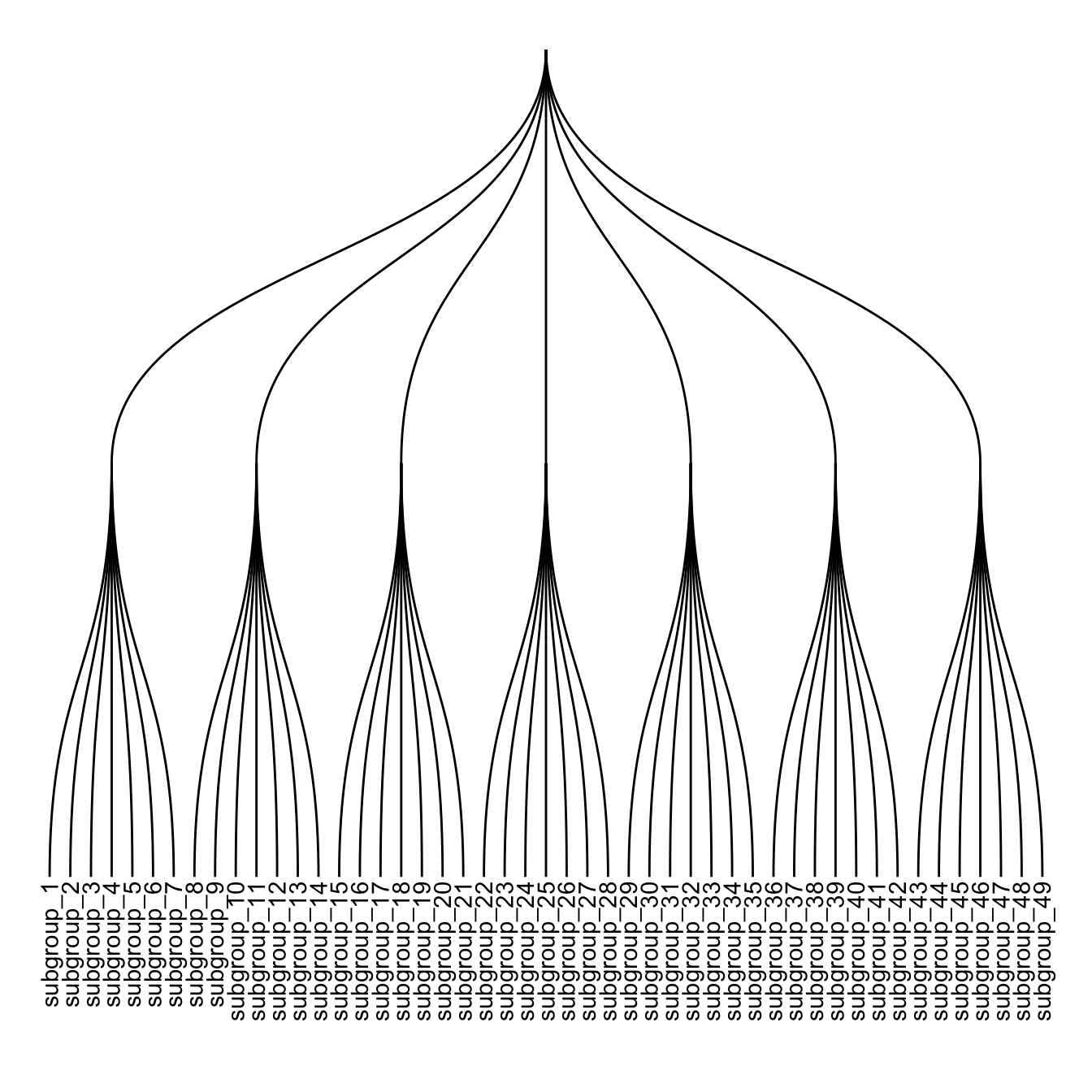# Dendrogram customization with R and ggraph

This post follows the previous introduction to `ggraph` and dendrogram. It shows how to customize the dendrogram: layout, edge style, node features and more.

Start by creating a dataset and a graph object using the `igraph` package.

``````# Libraries
library(ggraph)
library(igraph)
library(tidyverse)
theme_set(theme_void())

# data: edge list
d1 <- data.frame(from="origin", to=paste("group", seq(1,7), sep=""))
d2 <- data.frame(from=rep(d1\$to, each=7), to=paste("subgroup", seq(1,49), sep="_"))
edges <- rbind(d1, d2)

# We can add a second data frame with information for each node!
name <- unique(c(as.character(edges\$from), as.character(edges\$to)))
vertices <- data.frame(
name=name,
group=c( rep(NA,8) ,  rep( paste("group", seq(1,7), sep=""), each=7)),
cluster=sample(letters[1:4], length(name), replace=T),
value=sample(seq(10,30), length(name), replace=T)
)

# Create a graph object
mygraph <- graph_from_data_frame( edges, vertices=vertices)``````

# Circular or linear `layout`

First of all, you can use a linear or a circular representation using the circular option thanks to the `layout` argument of `ggraph`.

Note: a customized version of the circular dendrogram is available here, with more node features and labels.``````# Left
ggraph(mygraph, layout = 'dendrogram', circular = FALSE) +
geom_edge_diagonal() ``````
``````# Right
ggraph(mygraph, layout = 'dendrogram', circular = TRUE) +
geom_edge_diagonal()``````

# Edge style

Then you can choose between different styles for your edges. The `ggraph` package comes with 2 main functions: `geom_edge_link` and `geom_edge_diagonal`.

Note that the most usual “elbow” representation is not implemented for hierarchical data yet.``````# Left
ggraph(mygraph, layout = 'dendrogram') +
``````# Right
ggraph(mygraph, layout = 'dendrogram') +
geom_edge_diagonal()``````

# Labels and Nodes

You probably want to add labels to give more insight to your tree. And eventually nodes. This can be done using `the geom_node_text` and `geom_node_point` respectively.

Note: the label addition is a bit more tricky for circular dendrogram, a solution is suggested in graph #339.``````# Left
ggraph(mygraph, layout = 'dendrogram') +
geom_edge_diagonal() +
geom_node_text(aes( label=name, filter=leaf) , angle=90 , hjust=1, nudge_y = -0.01) +
ylim(-.4, NA)``````
``````# Right
ggraph(mygraph, layout = 'dendrogram') +
geom_edge_diagonal() +
geom_node_text(aes( label=name, filter=leaf) , angle=90 , hjust=1, nudge_y = -0.04) +
geom_node_point(aes(filter=leaf) , alpha=0.6) +
ylim(-.5, NA)``````

# Customize aesthetics

`ggraph` works the same way as `ggplot2`. In the aesthetics part of each component, you can use a column of your initial data frame to be mapped to a shape, a color, a size or other..``````ggraph(mygraph, layout = 'dendrogram') +
geom_edge_diagonal() +
geom_node_text(aes( label=name, filter=leaf, color=group) , angle=90 , hjust=1, nudge_y=-0.1) +
geom_node_point(aes(filter=leaf, size=value, color=group) , alpha=0.6) +
ylim(-.6, NA) +
theme(legend.position="none")``````

Related chart types

## Contact

This document is a work by Yan Holtz. Any feedback is highly encouraged. You can fill an issue on Github, drop me a message on Twitter, or send an email pasting yan.holtz.data with gmail.com.